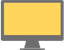Some test text!

# Search PDF files for text in Python

More languages

chevron_right
More languages
JavaScript
Java (Android)
C++
C#
C# (.NET Core)
Java
Kotlin
Obj-C
JS (Node.js)
PHP
Python
Ruby
Swift
C# (UWP)
VB
C# (Xamarin)

Sample Python code for using PDFTron SDK to search text on PDF pages using regular expressions. The TextSearch utility class builds on functionality available in TextExtractor to simplify most common search operations. Learn more about our Python PDF Library and PDF Indexed Search Library.

To run this sample, get started with a free trial of PDFTron SDK.

``````#---------------------------------------------------------------------------------------
#---------------------------------------------------------------------------------------

import site
import sys
from PDFNetPython import *

# This sample illustrates the basic text search capabilities of PDFNet.

# Relative path to the folder containing the test files.
input_path = "../../TestFiles/"
output_path = "../../TestFiles/Output/"

def main():
# Initialize PDFNet
PDFNet.Initialize()
doc = PDFDoc(input_path + "credit card numbers.pdf")
doc.InitSecurityHandler()

txt_search = TextSearch()
mode = TextSearch.e_whole_word | TextSearch.e_page_stop

pattern = "joHn sMiTh"

# call Begin() method to initialize the text search.
txt_search.Begin(doc, pattern, mode)

step = 0

# call Run() method iteratively to find all matching instances.
while True:
searchResult = txt_search.Run()
if searchResult.IsFound():
if step == 0:
# step 0: found "John Smith"
# note that, here, 'ambient_string' and 'hlts' are not written to,
# as 'e_ambient_string' and 'e_highlight' are not set.

print(str(searchResult.GetMatch()) + "'s credit card number is: ")

# now switch to using regular expressions to find John's credit card number
mode = txt_search.GetMode()
mode |= TextSearch.e_reg_expression | TextSearch.e_highlight
txt_search.SetMode(mode)
pattern = "\\d{4}-\\d{4}-\\d{4}-\\d{4}"     #or "(\\d{4}-){3}\\d{4}"
txt_search.SetPattern(pattern)
step = step + 1
elif step == 1:
# step 1: found John's credit card number
print("  " + searchResult.GetMatch())

# note that, here, 'hlts' is written to, as 'e_highligh' has been set.
# output the highlight info of the credit card number
hlts = searchResult.GetHighlights()
hlts.Begin(doc)
while hlts.HasNext():
print("The current highlight is from page: " + str(hlts.GetCurrentPageNumber()))
hlts.Next()

# see if there is an AMEX card number
pattern = "\\d{4}-\\d{6}-\\d{5}"
txt_search.SetPattern(pattern)

step = step + 1
elif step == 2:
# found an AMEX card number
print("\nThere is an AMEX card number:\n  " + searchResult.GetMatch())

# change mode to find the owner of the credit card; supposedly, the owner's
# name proceeds the number
mode = txt_search.GetMode()
mode |= TextSearch.e_search_up
txt_search.SetMode(mode)
pattern = "[A-z]++ [A-z]++"
txt_search.SetPattern(pattern)
step = step + 1
elif step == 3:
# found the owner's name of the AMEX card
print("Is the owner's name:\n  " + searchResult.GetMatch() + "?")

# add a link annotation based on the location of the found instance
hlts = searchResult.GetHighlights()
hlts.Begin(doc)

while (hlts.HasNext()):
cur_page = doc.GetPage(hlts.GetCurrentPageNumber())

i = 0
# assume each quad is an axis-aligned rectangle
x1 = min(min(min(q.p1.x, q.p2.x), q.p3.x), q.p4.x)
x2 = max(max(max(q.p1.x, q.p2.x), q.p3.x), q.p4.x)
y1 = min(min(min(q.p1.y, q.p2.y), q.p3.y), q.p4.y)
y2 = max(max(max(q.p1.y, q.p2.y), q.p3.y), q.p4.y)
i = i + 1
hlts.Next()
doc.Save(output_path + "credit card numbers_linked.pdf", SDFDoc.e_linearized)
break
elif code == TextSearch.e_page:
pass
else:
break

doc.Close()

if __name__ == '__main__':
main()``````
close

## Free Trial

Get unlimited trial usage of PDFTron SDK to bring accurate, reliable, and fast document processing capabilities to any application or workflow.

Select a platform to get started with your free trial.

Unlimited usage. No email address required.